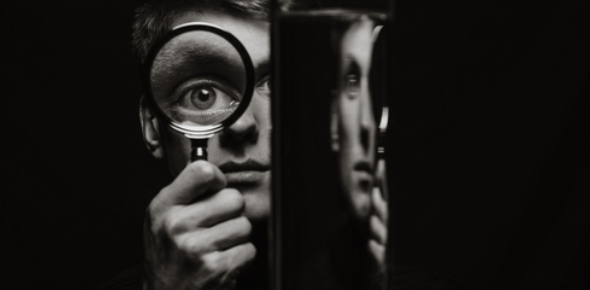# Reflection And Refraction Quiz

10 Questions | Attempts: 6657Settings• 1.
What is the normal?
• A.

The line perpendicular to the reflecting or refracting surface

• B.

The mean angle

• C.

The common angle at which waves reflect

• D.

How waves reflect and refract

• 2.
What is the angle of incidence?
• A.

The angle between the incident ray and the normal

• B.

The angle between the normal and the surface

• C.

The angle between the surface and the ray

• D.

The angle between the reflected ray and the normal

• 3.
What is the incident ray?
• A.

The ray before it hits the surface

• B.

The ray after it hits the surface

• C.

The ray after refraction

• D.

The ray at the normal

• 4.
What happens to the speed of light as it passes through a different medium?
• A.

It changes speed

• B.

The speed stays the same

• 5.
What happens when light is refracted?
• A.

It refracts towards the normal

• B.

The light stays the same

• C.

The normal changes

• D.

The light stays in the material

• 6.
What is a critical angle?
• A.

The largest angle of incidence for which refraction can still occur

• B.

The minimum angle for a normal

• C.

The angle of the emergent ray

• D.

The angle at which refraction occurs

• 7.
When light passes through a denser medium it speeds up.
• A.

True

• B.

False

• 8.
What is total internal reflection?
• A.

When light passing from a medium with a moderate refractive index into one with a low refractive index and completely reflects

• B.

When light reflects on a surface

• C.

Another name for the emergent ray

• D.

When a substance absorbs all the light

• 9.
Light can be refracted and reflected at the same time.
• A.

True

• B.

False

• 10.
What is a phenomenon that optical fibers uses?
• A.

Total internal reflection

• B.

Refraction

• C.

Diffusion

• D.

Reflection

## Related TopicsBack to top
×

Wait!
Here's an interesting quiz for you.